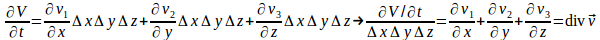## Proof That Loss of Fluid Per Unit Volume Equals Divergence of Velocity Field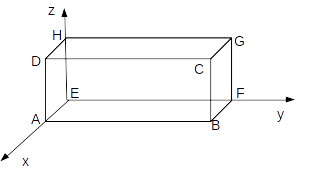The x component of the velocity at the centre of the face ABCD above is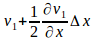The x component of the velocity at the centre of the face EFGH above is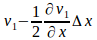The volume of fluid crossing ABCD per unit time is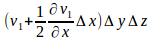The volume of fluid crossing EFGH per unit time is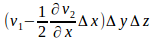The rate at which water is lost is the difference of these two and equals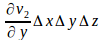Similarly the net loss of fluid in the y and z directions areand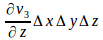restively.

Adding these gives the rate of loss of fluid overall as